# Logic and the philosophy of mathematics in the nineteenth century

Logic and the philosophy of mathematics in the nineteenth century
Logic and the philosophy of mathematics in the nineteenth century John Stillwell INTRODUCTION In its history of over two thousand years, mathematics has seldom been disturbed by philosophical disputes. Ever since Plato, who is said to have put the slogan ‘Let no one who is not a geometer enter here’ over the door of his academy, mathematics has been the standard of exact truth against which other philosophical discourse is measured. Descartes’ Discourse on Method grew out of his Geometry. Spinoza wrote his Ethics in the style of Euclid’s Elements. And Leibniz dreamed of a Characteristica Universalis by means of which ‘we should be able to reason in metaphysics and morals in much the same way as in geometry and analysis’. Mathematics is not only supremely logical, it is also astonishingly powerful. Here is how it struck Thomas Hobbes, according to Aubrey’s Brief Lives: He was 40 yeares old before he looked on Geometry; which happened accidentally. Being in a Gentleman’s Library, Euclid’s Elements lay open, and ‘twas the 47 El. libri I. He read the Proposition. By G——, sayd he (he would now and then sweare an emphaticall Oath by way of emphasis) this is impossible! So he reads the Demonstration of it, which referred him back to such a Proposition; which proposition he read. That referred him back to another, which he also read…that at last he was demonstratively convinced of that trueth. This made him in love with Geometry. ([9.1], 230) It was this power of mathematics to draw unexpected conclusions, to solve difficult problems, that overcame the occasional philosophical doubts about the validity of its methods. Up until 1800 the main doubts about mathematics centred on the following issues: the existence of irrational numbers; the use of numbers in geometry; the existence of imaginary numbers; infinite objects and processes. Irrational numbers were a thorn in the side of the Pythagoreans (c. 500 BC), who believed that the natural numbers 1, 2, 3…were the key to understanding the universe. They found, for example, that musical harmony involved simple natural numbers— halving the length of a vibrating string raised its pitch an octave, reducing its length to 2/3 raised its pitch a fifth, and so on. However, they also found the theorem of Pythagoras, which implies that the diagonal of the unit square is the square root of 2, √2, and then were horrified to discover that √2 is not a ratio of natural numbers. In their view, this meant √2 was not a number at all, and hence numbers were not an adequate measure of geometric quantities. Rather than abandon geometry, the Greeks developed a geometric system parallel to arithmetic in which lengths were added and multiplied like numbers. It was also possible to compare a length such as √2 with ratios of natural numbers (rational numbers). That is, one could say whether m/n< or √2 m/n> √2 for any natural numbers m, n. The geometric meaning of m/n< √2 can be seen from the equivalent relation m<n, √2 which says that the line segment consisting of m units of length is contained in the line segment consisting of n copies of √2. This occurs just in case m2<2n2. Thus the geometric relation m/n< √2 is in fact equivalent to the natural number relation m2<2n2. Eudoxus (c. 350 BC) realised that to know the position (> or <) of a length ℓ relative to arbitrary rational numbers m/n is to know ℓ with complete precision. Hence calculations in the world of lengths are just as exact as those in the world of natural numbers. If m/n<ℓ and m/n′<ℓ′, for example, then one can conclude that m/n+m/n′<ℓ +ℓ′, and in this way one knows all rational numbers less than ℓ+ℓ′. By such arguments, Eudoxus was able to develop a system of calculating with lengths and comparing them with (ratios of) natural numbers. It was preserved in Book V of Euclid’s Elements and became known as the theory of proportion. Eudoxus also developed a more general method for reasoning about geometric quantities called the method of exhaustion. Just as the theory of proportion captures an irrational by comparing it with arbitrary rationals, the method of exhaustion captures a figure of unknown magnitude by comparing it with known ones. Typically, a curve will be compared with polygons, and this may enable the exact determination of areas and volumes of curved figures when the corresponding polygonal ones are known. For example, Archimedes showed that the area A of the parabolic segment (Figure 1) is 4/3 that of the inscribed triangle Δ1 by showing It follows that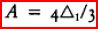because any value of A unequal to 4Δ1/3 can be refuted by summing sufficiently many triangles Δ1,…,Δn. The method ‘exhausts all possibilities’ exceptand, more importantly, each incorrect possibility is eliminated by reasoning about the finite sum of Δ1,…,Δn. Figure 1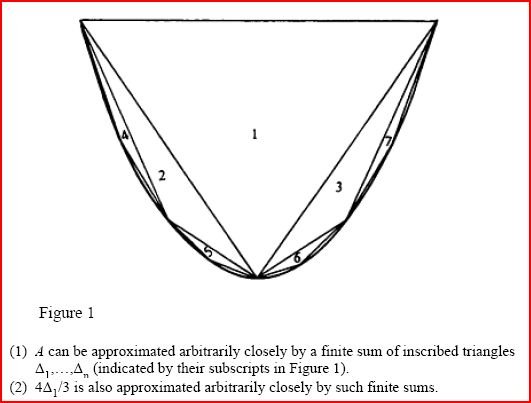The theory of proportions and the method of exhaustion enabled mathematicians to sidestep their first encounter with infinity. It was not necessary to sum infinitely many triangles to determine A, for example; the sum of Δ1,…,Δn was sufficient, provided n was arbitrary. One might say that infinity is present in the arbitrariness of n, but only ‘potentially’ so. The Greeks (and most mathematicians until the nineteenth century) drew a sharp distinction between the potential infinity which supplies natural numbers one by one, and the actual infinity which delivers them all at once. The concept of natural number is in fact potential infinity in a nutshell; the process of beginning with 0 and adding 1 ‘indefinitely’ is what the natural numbers are all about. The idea that this process could ever be completed seems at first as unnatural and uncalled for as a last natural number. However, the distinction between potential and actual infinity is not so easy to maintain in other cases. Consider the following example. To travel a distance of one kilometer, say, one first has to reach the 0.9 kilometer mark, then the 0.99 kilometer mark, then the 0.999 kilometer mark…, and in general the 1–1/10n kilometer mark for each n. One seemingly cannot complete the journey without also completing the infinity of values 1–1/10n. A similar example is discussed in Aristotle’s Physics, 239b, 14, under the name of Zeno’s paradox of Achilles and the tortoise. Aristotle refutes it by appealing to the (potentially) infinite divisibility of space and time. The kilometer can be subdivided at 0.9, 0.99, 0.999,…and the time interval required for the journey can be subdivided similarly, so completion of the journey involves only the potential infinity of subdivisions. We do not know the purpose of Zeno (c. 450 BC) in formulating his paradoxes, since they are known to us only through Aristotle, who wished to debunk them. Nevertheless, Zeno does seem to have put the fear of infinity into Greek mathematics. It is otherwise hard to understand the rejection of irrational numbers, and the restriction to potential infinity even in cases where actual infinity seems harmless. We now know, in fact, that Archimedes used actual infinity to discover his results on areas and volumes. His original methods were uncovered only in 1906, thanks to some extraordinary detective work by Heiberg ([9.26], 5). By then, it was too late for them to have any effect on mathematical practice. After the classical era, the next major encounter with infinity was in the seventeenth century. The occasion was the invention of the calculus, a resurgence of Archimedes’ theory of curved figures powered by the new algebraic geometry of Fermat and Descartes. Algebra made an enormous difference to geometry. Whereas Archimedes had to make an ingenious new approach to each new figure—for example, the approach to the parabolic segment using specially placed triangles—calculus dealt with a great variety of figures in the same way, via their equations. That was the whole point. Calculus was a method of calculating results, rather than proving them. If pressed, mathematicians could justify their calculations by the method of exhaustion, but it seemed impractical if not unnecessary to do so. Even before the stream of new results reached full flood with Newton and Leibniz (from 1665 onward) Huygens had warned: Mathematicians will never have enough time to read all the discoveries in Geometry (a quantity which is increasing from day to day and seems likely in this scientific age to develop to enormous proportions) if they continue to be presented in a rigorous form according to the manner of the ancients. (, 337) In fact, Huygens was probably the only major mathematician who stuck to the ‘methods of the ancients’. The methods of calculus were so much more powerful and efficient that rigour became secondary. Hobbes and Berkeley wrote scathing attacks on the illogical language of ‘infinitesimals’ used by mathematicians as a substitute for exhaustion, but they could not deny that it got results. And what results! By the middle of the eighteenth century, calculus had solved almost all the problems of classical geometry, and new ones the ancients had not dreamed of. It had also revealed the secrets of the heavens, explaining the motions of the moons and planets with uncanny precision. Apart from a few marginal controversies over such things as the infinite series 1−1+1−1+…, all the results of calculus stood up to rigorous scrutiny. The confidence inspired by the success of calculus was infectious. During the eighteenth century, mathematicians pushed their luck even further, using some concepts they did not know how to justify. They freely used √-1 while calling it ‘imaginary’ or ‘impossible’. They assumed that arbitrary continuous functions were expressible as infinite sums of sine waves. And once again their faith was rewarded, with an avalanche of new discoveries in mathematics and physics by d’Alembert, the Bernoullis, Euler and Lagrange. With this much success, mathematicians could afford to ignore philosophical questions about the meaning and rigour of their work. Only a direct contradiction in the heart of mathematics could give them pause. When it came, all the forgotten fears of irrationality and infinity would come back to the surface. THE CRISIS IN GEOMETRY Before 1800, geometry was thought to be part of physics. Its simplest elements—straight lines and circles—were idealizations of the simplest physical curves, constructed by the simplest drawing instruments, the straight edge and compasses. However, what set geometry apart from the rest of physics was its logical structure. It appeared that all properties of straight lines and circles (in the plane) were logical consequences of five axioms postulated by Euclid in his Elements (c. 300 BC): Let the following be postulated 1. To draw a straight line from any point to any point. 2. To produce a finite straight line continuously in a straight line. 3. To describe a circle with any centre and distance. 4. That all right angles are equal to one another. 5. That, if a straight line falling on two straight lines make the interior angles on the same side less than two right angles, the lines, if produced indefinitely, meet on that side on which are the angles less than the two right angles. ([9.27], 154) Euclid’s axioms were physically plausible, at least within the limits of measurement possible before 1800, though of course the fifth axiom could not be tested properly with finite lines. What was more important, in the opinion of most mathematicians and philosophers, was that no alternatives to Euclid’s axioms could be imagined. In particular, it seemed impossible to imagine either of the following alternatives to axiom 5: 5+. Two straight lines, if produced indefinitely, meet on both sides. 5. There are straight lines which fail to meet even though a straight line falling on them makes interior angles on the same side less than two right angles. (Or the following logical equivalent: given a line L and point P not on L there is more than one line through P not meeting L.) The strongest statement of the a priori nature of geometry was made by Kant in his Critique of Pure Reason. Kant believed that the fact that we cannot imagine alternatives such as 5+ and 5 made Euclid’s geometry the only one logically possible or meaningful: It is therefore, solely from the human standpoint that we can speak of space, of extended things, etc. If we depart from the subjective condition under which alone we can have outer intuition, namely, liability to be affected by objects, the representation of space stands for nothing whatsoever. ([9.31], 71) Kant’s philosophy was the high-water mark of intuitive geometry. The tide turned during the nineteenth century when mathematicians became increasingly absorbed with the construction of geometries contradicting Euclid, and later with the reconstruction of Euclidean geometry itself. These investigations stemmed from dissatisfaction with Euclid’s axiom 5, the so-called parallel axiom. Gauss, Bolyai and Lobachevsky realized that it was impossible to confirm the parallel axiom by physical experiment, and in fact conceivable that one could refute it. This was because the parallel axiom has the consequence that the angle sum (in radians) of any triangle is π. Since perfectly accurate measurement is impossible, one could never be sure that an angle sum measured to be π was in fact equal to π; and conceivably a triangle could be found with angle sum definitely not equal to π, i.e. differing from π by more than experimental error. While no physical refutation of the parallel axiom succeeded at the time (success was not achieved until 1919, as part of the confirmation of general relativity theory) its very possibility was enough to encourage Gauss, Bolyai and Lobachevsky to explore an alternative to Euclidean geometry. Their investigations were made independently, roughly between 1800 and 1830, and reached similar conclusions. Each of them studied the replacement of the parallel axiom by the axiom 5. The geometry that results from this replacement is now called hyperbolic geometry. It is in some ways more complicated than Euclidean geometry. For example, there are no similar figures of different sizes. There are no squares. Equilateral triangles of different sizes have different angles (always less than the angle π/3 of a Euclidean equilateral triangle). However, in other ways hyperbolic geometry is extremely elegant and convenient. The fact that equilateral triangles of different sizes have different angles yields an absolute unit of length. One could define the unit to be, say, the side of the equilateral triangle with angle π/4, since there is only one size of equilateral triangle with this angle. Likewise, the fact that the angle sum of a triangle is less than π makes it possible to show that the area of a triangle is proportional to the difference between π and the triangle’s angle sum. Thus, in hyperbolic geometry, area can be measured by angles. These beautiful consequences of axiom 5, and the non-appearance of any contradictory consequences, convinced Gauss, Bolyai and Lobachevsky that hyperbolic geometry was meaningful and worth pursuing. However, there was no immediate threat to the authority of Euclid and Kant. Gauss was too afraid of controversy to publish his results. Bolyai gave up soon after publication of his work in 1832, discouraged by the lack of response from other mathematicians and troubled by the possibility that contradictions might yet emerge. Lobachevsky published doggedly from the obscurity of Kazan from 1829 until 1856, the year of his death, without any encouragement from the outside world. (Gauss in fact admired Lobachevsky’s work, but communicated his feelings only to his friend Schumacher in a letter [9.24]. But in 1855 Gauss died, and his interest in hyperbolic geometry became known to the mathematical world through the release of his unpublished papers. This led to a more widespread interest in hyperbolic geometry, particularly in the light of differential geometry, a field also pioneered by Gauss with his book on curved surfaces [9.23], and invigorated by the ideas of Riemann [9.38] on curved space of arbitrary dimensions. On any smooth surface there are curves called geodesics which are ‘as straight as possible’ and hence can be regarded as the ‘lines’ of a ‘geometry’. On the sphere, for example, the geodesics are the great circles, because a great circle gives the shortest distance between any two points on the sphere. The corresponding ‘geometry’ is spherical geometry, which studies such things as spherical triangles. Spherical geometry had in fact been studied since ancient times because of its applications to astronomy and navigation, but the idea of its being a ‘geometry’ with theorems contrary to those of Euclidean geometry had not come up, perhaps because its ‘lines’ are manifestly finite and closed. However, the curved ‘lines’ on the sphere can be made straight by projecting the sphere from its centre on to a tangent plane (Figure 2). Admittedly, only half of each spherical ‘line’ can be seen at a time this way, and spherical distance becomes distorted. However, it does make spherical geometry look like a geometry of genuine lines, and it raises an interesting question. For which surfaces is there a map to the plane carrying geodesics to straight lines? Figure 2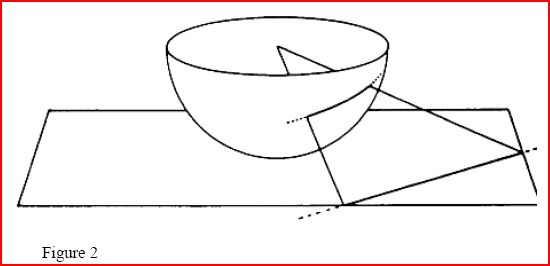Beltrami [9.2] found the answer in terms of the curvature of the surface, a concept introduced in Gauss [9.22]. The surfaces whose geodesics can be mapped to straight lines are precisely those of constant curvature. The plane itself is of zero curvature, a sphere has constant positive curvature—which explained the known examples—but there are also surfaces of constant negative curvature. The first of these to be discovered was the pseudosphere, a trumpet-shaped surface (Figure 3) whose geometry had been investigated by Gauss’s student Minding in 1840. Minding even made the pregnant discovery that its triangles are governed by formulas like those for spherical triangles, but with the circular functions sine and cosine replaced by their hyperbolic analogues sinh and cosh (hence the name ‘pseudosphere’). The same formulas had already been found to hold in hyperbolic geometry by Lobachevsky [9.34]. Figure 3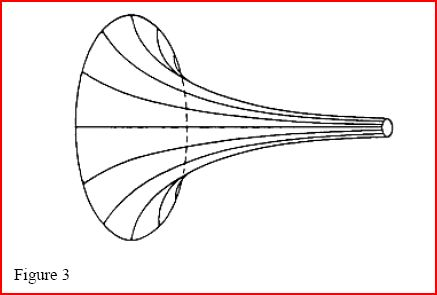Unfortunately the pseudosphere cannot be regarded as a complete realization of hyperbolic geometry, because ‘lines’ on the pseudosphere do not extend indefinitely. The rim of the trumpet is an impassable boundary, beyond which the pseudosphere cannot be extended because its curvature becomes undefined there. In 1868 Beltrami [9.3] saw a way round this difficulty with his mapping of geodesics to straight lines. The pseudosphere is mapped on to a portion of the disc (Figure 4) and the line segments representing its geodesics have a natural exten- sion to the whole interior of the disc. (The boundary of the disc is infinitely far away, in the sense of distance on the pseudosphere. In particular, the point E represents the infinitely distant end of the trumpet.) Thus the whole interior of the disc has a geometry, inherited from the pseudosphere, and it is the complete hyperbolic geometry of Gauss, Bolyai and Lobachevsky. The validity of the hyperbolic parallel axiom 5 is particularly clear, as Figure 5 shows. Figure 4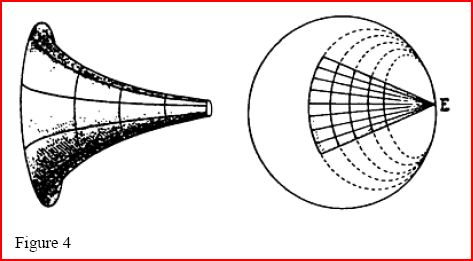Figure 5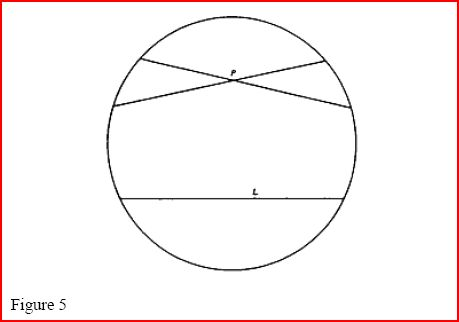Beltrami had found what we now call a model of hyperbolic geometry—an interpretation of the terms ‘point’, ‘line’, etc. within Euclidean geometry under which the axioms 1, 2, 3, 4, 5 are satisfied. It follows that the Gauss-Bolyai-Lobachevsky axioms cannot lead to a contradiction, unless there is a contradiction in Euclidean geometry itself. This was Beltrami’s great contribution to the philosophy of geometry. As he put it (rather modestly) in his 1868 paper: In recent times the mathematical public has begun to take an interest in some new concepts which seem destined, if they prevail, to change profoundly the whole complexion of classical geometry. These concepts are not particularly recent. The master Gauss grasped them at the beginning of his scientific career, and although his writings do not contain an explicit exposition, his letters confirm that he had always cultivated them and attest to his full support for the doctrine of Lobachevsky. …we have sought, to the extent of our ability, to convince ourselves of the results of Lobachevsky’s doctrine; then, following the tradition of scientific research, we have tried to find a real substrate for this doctrine, rather than admit the necessity for a new order of entities and concepts. We believe we have attained this goal for the planar part of the doctrine. (Beltrami 1868 in [9.17], 533) Beltrami’s model showed that Euclid’s axioms 1, 2, 3, 4, 5 are not the only logical possibility. It was therefore admissible to doubt that they were true of physical space. If Euclid’s geometry was not about physical space, what was it about? ARITHMETIZATION With the discovery of non-Euclidean geometry the nature and existence of geometric objects was called into question. It was time to take up an option which had been available for two centuries, but held back out of respect for the Greek tradition of separating the concepts of number and length—the arithmetization of geometry. Around 1630, Fermat and Descartes independently discovered the method of co-ordinates which makes it possible to describe curves by equations in variables x and y. Given two lines OX and OY in the plane (Figure 6), any point P is determined by its distances x and y from them. (It is convenient, though not necessary, to take OX and OY to be perpendicular, and to let y and x be the perpendicular distances from P to them.) As P traverses a curve in the plane, x and y enter a certain relationship, which Fermat and Descartes found they could easily describe in many important cases. Figure 6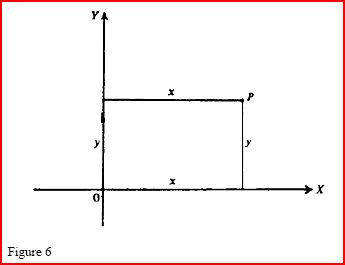For example, if P describes the straight line passing through O and equidistant from OX and OY, then y=x. More generally, any straight line is described by an equation of the form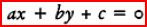where a, b, c are constants, and which we now call a linear equation for that reason. Conversely, any such equation (provided a, b are not both zero) represents a straight line, so there is a correspondence between lines and linear equations. Linear equations are recognizable algebraically as those in which x and y occur to the first power. The next simplest equations, called quadratic, include terms in x2, y2 and xy as well as linear terms. Fermat and Descartes discovered that the curves described by quadratic equations are precisely the conic sections (ellipses, parabolas, hyperbolas), which had been studied by the Greeks, particularly by Apollonius, c. 200 BC. Apollonius even knew the relationship between x and y for a conic section, but he expressed it in words (taking about half a page!) and not as an equation. Lacking algebraic notation, the Greeks could hardly form the idea of equations, let alone manipulate them so as to be able to recognize the curves they described. Fermat and Descartes had the advantage of a well developed notation and technique for algebra, thanks to the efforts of their compatriot Viète (1540–1603) and the Italians del Ferro, Tartaglia, Cardano, Ferrari and Bombelli in the sixteenth century. In turn, Descartes’ Geometry (1637, [9.15]) made a big impression on Newton, who carried the analysis of equations beyond the range of classical geometry with a classification of cubic curves [9.37]. Thus by the end of the seventeenth century it was well established that curves could be studied by algebra, at least the curves expressed by polynomial equations, i.e. equations formed by applying arithmetic operations to x and y. However, these developments did not prompt any reassessment of the nature of curves. No one said that curves were equations. Moreover, the variables x and y were regarded as lengths rather than numbers. Thanks to Eudoxus’ theory of proportions (see Introduction above), x and y could be regarded as lengths while only arithmetic operations were applied to them, so the Greek segregation of geometry from number remained in force. The inadequacies of the theory of proportions (and indeed the theory of numbers) were not exposed until questions about curves in general began to be asked in the eighteenth century. Perhaps the turning point was Gauss’s proof of the fundamental theorem of algebra [9.22]. The exact statement proved by Gauss does not concern us here (the modern equivalent is that any polynomial equation has a solution in the complex numbers). The point of interest is the following statement assumed by Gauss in his proof. If K1 and K2 are curves inside a circle, and the endpoints A1, B1 of K1 separate the endpoints A2, B2 of K2 on the circle (Figure 7), then K1 and K2 have a common point. The only justification Gauss offered for this statement was that ‘no one, to my knowledge, has ever doubted it’. This was probably true, but Figure 7nevertheless a poor excuse, particularly since Gauss was well aware of gaps in previous attempts to prove the fundamental theorem, and at pains to point them out. Quite likely, the only reason the statement had never been doubted is that it had never previously been used in a mathematical proof. Gauss’s proof was probably the first existence proof in the history of mathematics—one where the existence of a point was proved without a means of constructing it—and probably the first to use topological reasoning. The basic concept of topology, namely continuity, had not even been defined in 1799, and Gauss’s claim is not easy to prove even when the notion of continuous curve has been made clear. It seems that Gauss realised the seriousness of the gap in his proof, because in 1816 he offered another proof of the fundamental theorem of algebra in which the role of continuity was minimized. In his second proof he assumed only that if p(x) is a polynomial such that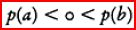for some real numbers a and b then p(c)=o for some c between a and b. This assumption, known as the intermediate value theorem (for polynomials) can be viewed as a special case of the assumption in the first proof, namely, where K1 is part of OX and K2 is part of the curve y=p(x) (Figure 8). Figure 8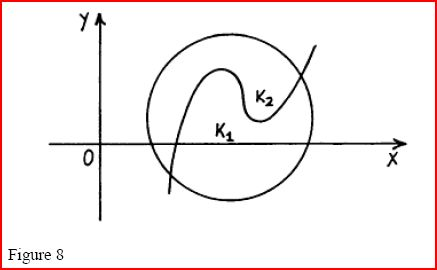The intermediate value theorem is needed even to show the existence of, which is the value for which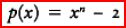becomes zero between, say, x=1 and x=2. Thus any proof of the fundamental theorem of algebra involves the intermediate value theorem, at least implicitly. This point was recognized by Bolzano in 1817 [9.5], immediately after the appearance of Gauss’s proof. Bolzano gave a proof of the continuity of polynomial curves y=p(x), and attempted a proof of the intermediate value theorem for arbitrary continuous curves y= f(x). His definition of continuity was essentially the modern one: a function fx varies according to the law of continuity for all values of x inside or outside certain limits means just that: if x is some such value, the difference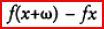can be made smaller than any given quantity provided ω can be taken as small as we please. (Bolzano 1817 in [9.17], 565) However, his proof of the intermediate value theorem assumes the following least upper bound property of real numbers: whenever a certain property M belongs to all values of a variable quantity i which are smaller than a given value and yet not for all values in general, then there is always some greatest value u, for which it can be asserted that all i<u possess property M ([9.17], 565) Actually, the value u is greatest lower bound of the values not having property M. It is now more usual to consider the equivalent least upper bound property: every bounded set of real numbers has a least upper bound. Bolzano was unable to prove this property rigorously because he did not have a definition of real number. Nevertheless, identifying the property was an important step. It set a requirement that any future definition of real number would have to meet, and was the first indication that further clarification of geometric concepts would depend on clarification of the concept of number. WHAT IS A REAL NUMBER In 1858, the last student of Gauss, Richard Dedekind, found himself teaching elementary calculus at the Polytechnic School in Zurich. He later wrote: In discussing the notion of the approach of a variable magnitude to a fixed limiting value, and especially in proving the theorem that every magnitude which grows continually, but not beyond all limits, must approach a limiting value, I had recourse to geometric evidences. Even now such resort to geometric intuition… I regard as exceedingly useful… But that this form of introduction into the differential calculus can make no claim to being scientific, no one will deny. ([9.14], 1) Dedekind, too, had glimpsed the elusive least upper bound property. He was determined to capture it. I made the fixed resolve to keep meditating on the question until I should find a purely arithmetic and perfectly rigorous foundation for the principles of infinitesimal analysis. The statement is so frequently made that the differential calculus deals with continuous magnitude, and yet an explanation of this continuity is nowhere given; even the most rigorous expositions of the differential calculus do not base their proofs on continuity…they either appeal to geometric notions…or depend upon theorems which are never established in a purely arithmetic manner. Among these, for example, belongs the abovementioned theorem, and a more careful investigation convinced me that this theorem, or any one equivalent to it, can be regarded in some way as a sufficient basis for infinitesimal analysis ([9.14], 7) Having seen clearly what the difficulty was: ‘It then only remained to discover its true origin in the elements of arithmetic and thus at the same time to secure a real definition of the essence of continuity. I succeeded Nov. 24, 1858’ ([9.14], 2). What then was Dedekind’s definition that captured the essence of continuity? In a nutshell, it was what Eudoxus would have said if he had believed in actual infinity. Like Eudoxus, Dedekind determined a length by comparison with rational numbers, but, unlike Eudoxus, Dedekind considered the totality of rational numbers to be an actual object—a set—and he declared the length being determined to be a number. The length √2, for example, is greater than a positive rational m/n if and only if 2n2>m2, as we observed in the Introduction above. This gives a numerical process for comparing m/n with √2, essentially Eudoxus’ theory of proportions. Dedekind sought a numerical object he could identify with √2, and found it in the completed outcome of the comparison process, namely the pair of sets of positive rationals \m/n : 2n2 < m2 and \m/n:2n2 > m2 In general, Dedekind defined a real number to be a partition of the rationals into two sets A1, A2 such that each member of A1 is less than every member of A1. He called such a partition a cut. Each rational number r itself produces a cut, or rather two cuts (one with r the maximum member of A1, the other with r the minimum member of A2), so the rationals are naturally absorbed into this more comprehensive concept of number. The real numbers also inherit natural operations of sum, product, etc. from the rational numbers. For example, if α is the cut (A1, A2) and (β is the cut (B1, B2) then α + β is the cut (C1, C2), where C1 is the set of sums a1+b1 where a1 is in A1 (i.e. a1 < α) and b1 is in B1 (i.e. b1 < β), and C2 consists of the rationals not in C1. In this way it becomes possible to prove results such as √2√3=√6, which Dedekind believed had not been proved rigorously before. (It would, however, have been possible to prove √2√3=√6 rigorously for lengths √2√3=√6 by the theory of proportions.) Finally, it was easy to prove the least upper bound property. If a real quantity α grows continually but not beyond a limit β say, consider the set L1 of all rationals belonging to lower sets A1 of values of α. Then if L2 is the set of rationals not in L1 (in particular L2 includes the rationals greater than β) it is easily seen that (L1, L2) is a cut and it is the least upper bound of the variable quantity α. The least upper bound property is one of several equivalent statements of what Dedekind called the continuity of the real numbers (nowadays called the completeness of the real numbers). Another is that there is no gap, that is, no partition of the real numbers into sets A1, A2 with each member of A1 less than every member of A2, no maximum member of A1 and no minimum member of A2. This property is an almost immediate consequence of Dedekind’s definition, which essentially fills each gap in the rational numbers by a real number. As Dedekind pointed out, the continuity of the real numbers enables them to serve as a model of points on the line. Thus the key step in the arithmetization of geometry was finally achieved. With the line defined as the set of real numbers x, it is natural to define the plane as the set of ordered pairs of real numbers, (x, y), and then curves can be defined by equations in x and y. This programme was completed in the Grundlagen der Geometrie of Hilbert (1899, [9.26]). Incidentally, the pair (x, y) is also used as a concrete representative of the complex number x+y√-I, thus giving a meaning to ‘imaginary’ numbers (see Introduction above). In the meantime, several other definitions of real number had been proposed, notably by Meray [9.32], Weierstrass [9.29] and Cantor [9.9]. Weierstrass [9.37] also gave the first rigorous proofs of the fundamental theorems on continuous functions, in particular the intermediate value theorem anticipated by Bolzano [9.5]. Meray, Weierstrass and Cantor all defined real numbers as sets of convergent infinite sequences—definitely a more complicated approach than Dedekind’s, but one more suited to modern analysis, where convergent sequences (of objects which are not necessarily numbers) are the stock in trade. All these definitions are equivalent, as it happens, so it does not matter which one is used to define the line. The important question is whether it is necessary to accept completed infinities to arithmetize the concept of line. With hindsight, one can see how the cut defining √2, say, might be explained away as a potential infinity (see the next section). But can the infinity of real numbers be regarded as merely potential? This was one of the profound questions answered by Cantor in the next episode of our story—the development of set theory. SETS Cantor, like Dedekind, was drawn into accepting actual infinities in order to clarify the meaning of points on the line. In Cantor’s case the problem was more technical (though with a pedigree going back to the Pythagorean investigation of vibrating strings), concerning the points of discontinuity of infinite sums of sine waves [9.9]. He found that such a set of points could be very complicated. So complicated, in fact, that an operation he used to ‘thin’ the set could be repeated infinitely often without removing all of it. He therefore decided to apply his thinning operation ‘more than an infinite number of times’, or rather, to continue applying it after the first infinity of applications was complete. The implication was, of course, that this infinity of operations was actual. To count the number of applications of the thinning operation, Cantor [9.11] introduced ordinal numbers, a generalization of natural numbers to infinity and beyond. The first infinite ordinal number, following the complete series 0, 1, 2, 3,…, is called ω. It is followed by ω+1, ω+2, ω+3,…, and this second infinity of ordinal numbers is followed by a number called ω'2. Any ordinal number α, like a natural number, has a successor α+1. Cantor [9.11] called this the ‘first principle of generation’ of ordinal numbers. He also introduced a second principle, which generates, ω, ω'2 and further ordinals by wholesale actualization of infinities: If any definite succession of defined whole real [i.e. ordinal] numbers exists, for which there is no largest, then a new number is created, by means of this second principle of generation, which is thought of as the limit of these numbers, i.e. it is defined as the next number larger than all of them. ([9.11], 196) In calling his ordinal numbers whole real numbers Cantor wished to stress their reality, i.e. the reality of infinite sets, rather than any analogy with the real numbers (which is reell in German and not the word real used by Cantor). There is a much better analogy with the natural numbers, of course, though it is striking that the ordinals share the least upper bound property with the real numbers. The relationship between the ordinals and the real numbers was in fact an unsolved problem of Cantor’s set theory, the continuum problem, and it remains today one of the great mysteries of mathematics. As will become clearer below, the real numbers are generated by a principle which is possibly even more powerful than Cantor’s second principle of generation for ordinal numbers. Cantor’s investigation of sets of real numbers led him to another generalization of the concept of natural number, the concept of cardinal number. Two sets A and B are said to have the same cardinal number if there is a one-to-one correspondence or pairing between the members of A and the members of B. The cardinal number of a finite set A is simply the (natural) number of members of A, because if A has, say, seventeen members then any set B whose members can be paired with the members of A will also have seventeen members. The set of all natural numbers has an infinite cardinal number which Cantor called ℵ₀ Cantor found that many seemingly ‘larger’ × sets can be put in one-to-one correspondence with the natural numbers, and hence also have cardinal number ℵ₀. One such set is the rational numbers. Another, as Cantor [9.10], found, is the algebraic numbers. A number is called algebraic if it is the solution of an equation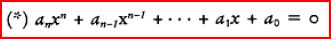where a0, a1,…, an are integers. Cantor observed that if each such equation is assigned the ‘height’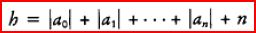then there are only finitely many equations with height h less than a given natural number i. It is therefore possible to make a (potentially infinite) list consisting of all equations of height ≤ 1, then all equations of height ≤ 2, then all equations of height ≤ 3…, thus obtaining a list of all equations (*), in which each equation has some natural number position j. This puts the equations (*) in one-to-one correspondence with the natural numbers j. Finally, if each equation is replaced by the (finite) list of its solutions we get a list of all algebraic numbers, on which each algebraic number appears at some natural number position k. The listing of the algebraic numbers is a very strong result, since it includes listings of many other sets, in particular the integers and the rational numbers. It follows that all these sets have cardinal number ℵ₀. However, it does not follow that the set of all real numbers has cardinal number ℵ₀. It was already known, from the work of Liouville [9.33], that certain real numbers are not algebraic. Cantor [9.10] discovered the more dramatic result that almost all real numbers are not algebraic. He showed that the cardinal number of the reals is not ℵ₀, by showing that the reals cannot be paired with the natural numbers. This result is known as the non-denumerability or uncountability of the set of real numbers. Non-denumerability was so unprecedented that Cantor initially attempted to downplay it as far as possible, calling his paper ‘On a Property of the Collection of All Real Algebraic Numbers’ and using non-denumerability only to give a new proof of Liouville’s result. (The algebraic numbers have cardinal number ℵ₀, the reals do not, so not all reals are algebraic, QED.) He soon realized, however, that non-denumerability was the gateway to a new world. It revealed the existence of cardinal numbers beyond ℵ₀, so mathematics was able to distinguish different kinds of infinity. It also showed that the real numbers could not be explained away as a potential infinity. For what is a potential infinity but one whose members can be paired with the natural numbers? As pointed out in the Introduction above, the natural numbers 0, 1, 2,…are the epitome of a potential infinity—a list which includes any given member, if carried sufficiently far. The non-denumerability of the reals means that any proposed listing of real numbers is doomed to incompleteness—certain numbers (depending on the list, of course) will never appear on it, no matter how long the list is continued. In 1891 [9.12] Cantor gave a second proof of non-denumerability which makes the futility of listing real numbers crystal clear. Suppose that x1, x2, x3,… is a list of real numbers, and let xi be expanded as a decimal up to and including the ith decimal place. For example, the list may begin like this: x1=17.7..., x2=0.16..., x3=2.131..., x4=0.7474..., x5=11.11312 … No matter how the list continues, a number x not on it may be constructed by making x different from x1 in the first decimal place, different from x2 in the second decimal place, different from x3 in the third decimal place…, and so on. An explicit way to do this (which avoids getting an expansion which is ultimately all 9s or all 0s, and hence expressible in two ways) is to let the ith decimal place of x be 1 if the ith decimal place of x1, is not 1, and otherwise let it be 2. Thus no list includes all the real numbers. Since the number x is constructed from the sequence of digits along the diagonal of the list, this technique came to be known as the diagonal argument. It was actually used before Cantor by du Bois Reymond [9.16], though in a less transparent way. Cantor [9.12] was the first to see that the diagonal argument was not simply a trick with numbers but actually a fundamental insight into the relationship between sets and their subsets, showing that any set has more subsets than members. This yields the astonishing conclusion that there is no largest set. To apply the diagonal argument in the general case let S be any set, and let Sx be a sub-set paired with element x. (You may like to think of Sx laid out in a ‘row’ beside element x, which is in a ‘column’ of the members of S.) Then one sub-set of S which is not among the sets Sx is the ‘diagonal sub-set’ D defined as follows: x belongs to D if and only if x does not belong to Sx. This ensures that D is different from each Sx, with respect to the element x in fact, and hence there are more sub-sets of S than members of S. The operation of forming all subsets of a given set is called the power set operation. This is the operation, mentioned above, which is possibly more powerful than the second principle of generation of ordinal numbers. While the generation of ordinal numbers admits a larger number only when there is some way of ‘approaching’ it, the power set operation creates a new set without offering any means of approach. In the case where S is the set of natural numbers it is easy to show (though a little technical) that the power set of S has the same cardinal number as the set of real numbers. Thus the continuum problem, which asks how big an ordinal number is required to count the real numbers, is about the size of the power set of the natural members. WHAT IS A NATURAL NUMBER? With the help of the set concept, Dedekind had reduced the concept of real number to the concept of rational number, as explained on pp. 255–7. Since relations between rational numbers are equivalent to relations between natural numbers, this meant that all the concepts of geometry and analysis were definable in terms of sets and the natural numbers 0, 1, 2, 3,… Most mathematicians felt that this was as solid a foundation as it was necessary or possible to have. After all, statements about the natural numbers were not contested (the way the parallel axiom, or the intermediate value theorem had been, for example) and no simpler concept than natural number seemed likely as a basis for mathematics. In taking this view, mathematicians tended to overlook the fact that the foundation was not just the natural numbers, but natural numbers and sets. Sets were unavoidable, as experience with the real numbers made clear, so if there was a simpler basis for mathematics than natural numbers and sets it had to be just sets. The first to grasp this possibility was Gottlob Frege, who developed a definition of natural numbers in terms of sets, or more precisely in terms of properties, in his 1884 book Die Grundlagen der Arithmetik [9.19]. There is a subtle but important difference between sets and properties (or concepts, as Frege called them). Corresponding to each set S there is the property of belonging to S, and for each property P there is the extension of P, consisting of all things with property P (or of all things which fall under concept P, as Frege put it). However, the extension of a property can have paradoxical behaviour if one assumes it to be a set (see pp. 266–8). This was not known in 1884, so there is no distinction between sets and extensions of properties in the Grundlagen. To make a distinction possible, and at the same time to give a concise description of Frege’s original idea, we shall use the term class for the extension of a property. Frege began with a highly entertaining demolition of previous attempts to define the notion of number, then opened his own investigation by defining what it means for two classes to have the same number. Like Cantor, he said that this is the case when there is a one-to-one correspondence between their members, though he pointed out that for finite classes this criterion had already been given by Hume (A Treatise of Human Nature, Book I, part III, section I). Note that it is not necessary to use the number 1 to define one-to- one correspondence. A relation ϕ(x,y) between the elements x of a class X and the elements y of a class Y is a one-to-one correspondence if for each x in X there is a y in Y such that ϕ(x,y), for each y in Y there is an x in X such that ϕ(x,y), if x≠x' and ϕ(x,y) and ϕ(x',y') then y≠y', if y≠y' and ϕ(x,y) and ϕ(x',y') then x≠x' The number of a class X may then be defined as the property of admitting a one-to-one correspondence with X, i.e. the class of all classes that admit a one-to-one correspondence with X ([9.19], 79). Frege spent some time discussing the numbers 0 and 1. Any contradictory property serves to define a class with number 0, and Frege used the property ‘not identical with itself’. Thus 0 is the class of classes admitting a one-to-one correspondence with the (empty) class of things not identical with themselves. The concept of the empty class was novel, and Frege felt he would have to overcome some resistance to the idea: Some may find it shocking that I should speak of a concept in this connection. They will object, very likely, that it contains a contradiction and is reminiscent of our old friends the square circle and the wooden iron. Now I believe that these old friends are not so black as they are painted. To be of any use is, I admit, the last thing we should expect of them; but at the same time, they cannot do any harm, if only we do not assume that there is anything that falls under them. ([9.19], 87). Frege proceeded to show that the empty class is not only harmless, but wonderfully fertile, by pulling the natural numbers out of it, one by one. First 1 is the number which belongs to the concept ‘identical with 0’. ([9.19], 90) That is, 1 is the class of classes which admit one-to-one correspondence with the class whose member is 0. Then to define n+1, we shall choose the concept ‘number of the series of natural numbers ending with n’. ([9.19], 92) That is, n+1 is the class of classes which admit one-to-one correspondence with the class whose members are 0, 1,…, n. Admittedly, there is something to be proved before we know that ‘the series of natural numbers ending with n’ is well defined. However, this was a matter of pure logic, which Frege already understood. As we shall see in the next section, he had laid the foundations of mathematical logic in 1879. Thus Frege’s work in 1884 was essentially the last step in a reduction of mathematics to pure logic, the previous steps being the definition of the real numbers in terms of rationals and the arithmetization of geometry and analysis. This stunning achievement went unnoticed at the time, even by Frege himself. Strange as it may seem, Frege rejected the arithmetization of geometry, so he missed the opportunity to extend his ideas beyond the field of number. When Hilbert’s Grundlagen der Geometrie [9.28] appeared in 1899, Frege wrote him several long letters ([9.20], 34) protesting against Hilbert’s definitions of ‘point’ and ‘line’ and insisting on spatial intuition as the source of geometric axioms. He seemed oblivious to all that had happened in geometry since Kant. The opportunity Frege missed was taken up by Bertrand Russell (with due credit to Frege) only after the turn of the century. WHAT IS LOGIC? Logic has been a part of mathematics since ancient times, but until the nineteenth century it was the least understood part. In mathematics there was no ‘theory’ of logic the way there was a theory of numbers, for example, no attempt to analyse the content of logic and to describe it completely. It is true that Leibniz and others had dreamed of making logic as exact and mechanical as calculation, but little progress resulted from these speculations. The proper understanding of logic arose from the attempt to understand the foundations of mathematics, and eventually encompassed it. The first important, though small, step was taken by George Boole. In his Mathematical Analysis of Logic (1847, [9.6]) and The Laws of Thought (1854, [9.7]) he introduced a logic of classes which is now known as Boolean algebra. In a deliberate imitation of algebraic notation, he used x, y, z,…to denote classes, and let xy, x+y and xy denote intersection, union and relative complement respectively. Since a thing belongs to xy if and only if it belongs to x and to y, to x+y if and only if it belongs to x or to y, to xy if and only if it belongs to x and not to y, the algebra of Boole’s product, sum and difference reflects the logic of ‘and’, ‘or’ and ‘not’, or propositional logic as it is now called. This algebra is not exactly the same as ordinary algebra—for example, x2= x for any class x—but it was near enough for Boole to venture the opinion that There is not only a close analogy between the operations of the mind in general reasoning and its operations in the particular science of Algebra, but there is to a considerable extent an exact agreement in the laws by which the two classes of operations are conducted. ([9.7], 6) He was correct in thinking that his algebra was similar to ordinary algebra in its operations and laws, but quite mistaken in thinking that such an algebra could reflect ‘general reasoning’. If thought was really as simple as Boole’s algebra we should not be able to grasp very much mathematics, not even the concept of number, as Frege realized in 1879. By reflecting on this difficulty, Frege was able to discover a vastly more powerful system of logic. The difference between Boole and Frege was that Boole was looking for a mathematics of logic, whereas Frege was looking for a logic of mathematics. Boole’s search was less fruitful, because the mathematics of his time contained a reflection of only a small part of logic—the logic of ‘and’, ‘or’ and ‘not’. We can now see that Frege made a better choice, but still it is astounding that he was able to succeed so completely at his first attempt. Frege’s logical system came to life fully grown in his Begriffsschrift (Concept Writing) of 1879 [9.18]. Unlike the Grundlagen of 1884, the Begriffsschrift is not a masterpiece of clear and persuasive writing; in particular, most of its logical content is expressed in a strange, labyrinthine symbolism of Frege’s own invention. Frege believed that the structure of deduction is best revealed by displaying it in a two-dimensional, treelike form. He managed to overcome his printer’s objections to this typographical nightmare, but apparently forgot that readers, too, prefer symbols to follow one another in a sequence. Fortunately it is now possible to translate the Begriffsschrift into a widely accepted notation, and I shall do so in what follows. The first difference one sees between Frege and Boole is that Frege regards the laws of logic not as algebraic identities but as theorems derivable from axioms by certain rules of inference. In the part of Frege’s logic that coincides with Boole’s, namely prepositional logic, the difference shows itself as follows. Instead of Boole’s ‘and’, ‘or’ and ‘not’, Frege uses ‘if…then’ and ‘not’, which we shall denote by ➙ and \Logic and the philosophy of mathematics in the nineteenth century respectively. This difference is not really important, since ‘and’, ‘or’, ‘not’ are expressible in terms of ‘if… then’, ‘not’ and vice versa, but ‘if…then’ and ‘not’ more naturally reflect the processes of deduction. In terms of ➙, \Logic and the philosophy of mathematics in the nineteenth century, Frege’s axioms are: It is one of the expository defects of the Begriffsschrift that Frege does not announce his axioms. He does say, at the beginning of his section II, that ‘a small number of laws’ (the axioms) ‘contain all of them’ (the theorems), but the reader finds the axioms only by searching through a long series of sample derivations. His rules of inference have to be found the same way; they are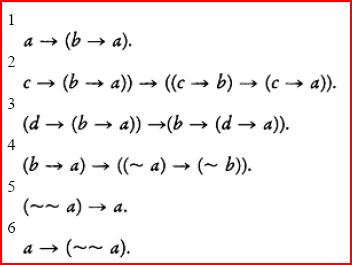Modus ponens: from theorems B and B B➙A A derive the theorem A (for any formulae A, B). Substitution: from theorem A derive the result of substituting, for each occurrence of a variable x in A, a formula ϕ(x). Since it is very easy to confuse a rule of inference with a theorem of logic—for example, modus ponens can be confused with the theorem b➙((b➙a)➙a) —Frege let his readers down badly by not making these rules explicit in section II. It seems clear that he did understand the difference between a theorem and a rule of inference. In his section I he discusses the idea of a ‘mode of inference’ and mentions modus ponens in particular. However, this was not clear enough for the logicians of his time, and they were still confused thirty years later (see, for example, the indiscriminate mixture of axioms, rules of inference and rules for the construction of formulae in Whitehead and Russell’s Principia Mathematica, vol. I, part I, section A*1 [9.41]). Frege offers no explanation for his claim that all laws of logic are consequences of his axioms. In fact for prepositional logic this is not difficult to prove, though proofs did not appear until around 1920. The claim is much more remarkable for his system as a whole, which is a logic of properties and relations now known as predicate logic. (Here ‘predicate’ is used as a synonym for ‘relation’, and a property is a special case of a relation.) The consideration of relations is the second, and major, difference between Frege and Boole. As we know from his analysis of the concept of number (pp. 261–3 above), Frege recognized that reasoning about properties and relations was fundamental to mathematics. He therefore decided to build the formulae of his logic from atomic formulae such as P(x) x has property P’ R(x, y) x and y are in relation R’ etc. All mathematical statements can be built from such atomic formulae with the help of the equality sign, =, the universal quantifiers, ∀ x (‘for all x’), ∀ y (‘for all y’), etc., and the connectives , \Logic and the philosophy of mathematics in the nineteenth century of prepositional logic. The task then was to find all the laws of this logic. Frege claimed that they could be obtained as theorems from the prepositional axioms 1–6, plus the following rules and axioms specific to predicate logic. ➙ introduction rules: from theorem A(x) derive ∀; from theorem ∀xA(x) derive B➙A(x) (when x does not occur in B). Axioms: 7B ➙ ∀xA(x), where f is any formula 8 c=c 9 (c=d) ➙ (f(c) ➙ f(d)), where c is any letter not in f. Quite amazingly, he was right! However, this is a deep theorem, first proved by Gödel [9.25], and it is doubtful that Frege fully understood the meaning of his claim, let alone knew why it was true. It was enough that he had captured logic in a system, of axioms and rules of inference—proving theorems about such a system was a task for a later generation of logicians. A FEW CLOUDS ON THE HORIZON In the last decades of the nineteenth century, mathematicians enjoyed a period of satisfaction with the foundations of their discipline, rather like that enjoyed by their colleagues in physics (before the discovery of relativistic and quantum effects). Geometry and analysis had been reduced to the theory of real numbers, the real numbers had been reduced to sets of natural numbers, and nearly everybody was satisfied with the natural numbers. If Frege’s work had been generally known the satisfaction could have been even greater (despite Frege’s personal objection to the arithmetization of geometry), because everything was wrapped up in his logic of classes. However, few were granted a glimpse of this mathematical heaven, because Frege remained in obscurity until the turn of the century. Then his logic became generally known only because Bertrand Russell found something wrong with it. The background to Russell’s discovery was this. In 1891 [9.12] Cantor introduced the general diagonal argument which shows that any set S has more sub-sets than members, as we saw on pp. 257–61 above. This obviously implies that there is no largest set, but it has a more serious implication: there is no set of all sets. If there were, it would be the largest set, by definition, yet the set of its sub-sets would be larger. Thus it is contradictory to suppose that the class of all sets is itself a set. This was the first of the socalled ‘paradoxes’ of set theory. A similar paradox, involving the class of all ordinal numbers, was published by Burali-Forti in 1897 [9.8]. Cantor did not discuss the paradoxes in print, but he suggested a possible resolution of them in a letter to Dedekind in 1899. a multiplicity can be such that the assumption that all of its elements ‘are together’ leads to a contradiction… If on the other hand the totality of elements of a multiplicity can be thought of without contradiction as ‘being together’, so that they can be gathered together into ‘one thing’, I call it a consistent multiplicity or a ‘set’. ([9.13], 14). In other words, to avoid contradictions, some classes should not be regarded as sets. This in fact is the solution favoured today, but at the time it was not clear how to recognize the inconsistent sets. Russell’s contribution was an analysis of the ‘set of all sets’ which made its inconsistency more obvious. Recall from pp. 257–61 how the diagonal construction is applied to a set S, with members x and sub-sets Sx paired with the members. One constructs the diagonal sub-set D of members x such that x does not belong to Sx. Then D differs from each Sx with respect to x. Now if S is the set of all sets x, then each subset y of S is also a member of S and we can pair y (as a sub-set) with itself (as a member). The diagonal set D then consists of all sets y such that y does not belong to y. Consequently, D differs from each set y with respect to the member y: y belongs to D if and only if y does not belong to y. But D is itself a member y of the set S of all sets, so D has the contradictory property of being different from itself. This is how Russell came to discover the inconsistency of the set D whose members are the sets that do not belong to themselves. Of course, its inconsistency is obvious once one has thought of it. Each of the two possibilities (1) D belongs to D, (2) D does not belong to D, implies the other. However, it is not easy to see how to prevent logic from being infected by such inconsistencies, and for this reason Russell’s discovery was a severe blow to Frege (see Russell [9.39], Frege [9.21]). Most of the classes Frege used to define numbers were inconsistent when regarded as sets, but if the logic of sets did not apply to them, what did? Evidently logic would have to be overhauled, taking the properties of sets into account, if inconsistencies were to be avoided. Even if the paradoxical sets were successfully excluded, how could one be sure that inconsistencies would not later arise in ‘indispensable’ parts of mathematics, such as arithmetic and analysis? In view of the trouble arising from the diagonal construction, one had to be suspicious of any field in which it was applicable, such as the theory of real numbers. Drastic cures for these ills were proposed by several mathematicians, especially those who had not gone along with set theory in the first place. Kronecker refused to accept completed infinities, and even denied the existence of irrational numbers. Poincaré and Borel opposed the use of ‘self-referential’ definitions. However, Hilbert probably spoke for the majority when he urged the construction of foundations to save as much as possible of set-theoretic mathematics. In 1900 [9.29] he presented a list of twenty-three problems to the mathematical community as tasks for the coming century. The first two were concerned with foundations, and focused on the real numbers: 1 The continuum problem: decide whether each infinite set of real numbers admits a one-to-one correspondence either with the set of natural numbers, or with the set of all real numbers. 2 The consistency of arithmetic: prove that no contradiction can arise from the axioms of arithmetic. (These axioms are essentially the basic properties of +, × and < normally assumed for real numbers, together with the least upper bound property.) These two problems effectively exposed the two greatest difficulties in the nineteenthcentury reduction of mathematics to logic. Firstly, the set concept was not clear. Between the simplest infinite set (the set of natural numbers) and the set of all its sub-sets (equivalently, the set of real numbers) was a gulf whose size and general nature was not understood. Secondly, it was not clear that mathematical concepts were correctly captured by axioms. The consequences of a given set of axioms were difficult to foresee and they could conceivably include contradictions. The answers to Hilbert’s problems 1 and 2 obtained so far do not boil down to a simple yes or no. They reveal many aspects of the foundations of mathematics not anticipated in the nineteenth century. The picture is no longer as simple as it once seemed, nevertheless the general outline remains intact. BIBLIOGRAPHY 9.1 Aubrey, J. Aubrey’s Brief Lives, Harmondsworth: Penguin, 1982. 9.2 Beltrami, E. ‘Risoluzione del problema: “Riportare i punti di una superficie sopra un piano in modo che le linee geodetiche vengano rappresentate da linee rette”’, Opere Matematiche, Milan, 1865:1 262–80. 9.3——‘Saggio di interpretazione della geometria non-euclidea’, Opere Matematiche, Milan, 1868, 1:374–405. 9.4 Bolyai, J. Scientiam spatii absolute veram exhibens, Appendix to W.Bolyai, Tentamen, Marosvásárhely 1832. English translation in R.Bonola, Noneuclidean geometry, New York: Dover, 1955. 9.5 Bolzano, B. Rein analytischer Beweis des Lehrsatzes dass zwischen je zwey Werthen, die ein entgegengesetzes Resultat gewähren, wenigstens eine reelle Wurzel der Gleichung liege (1817), Ostwalds Klassiker, vol. 153, Leipzig: Engelmann, 1905. 9.6 Boole, G. The Mathematical Analysis of Logic, London and Cambridge, 1847. 9.7——An Investigation of the Laws of Thought, London, 1854. 9.8 Burali-Forti, C. ‘Una questione sui numeri tranfiniti’, Rendiconti del Circolo matematico di Palermo, 11 (1897):154–64. English translation in From Frege to Gödel, ed. J.van Heijenoort, Cambridge, Mass.: Harvard University Press, 1967:104– 11. 9.9 Cantor, G. ‘Über die Ausdehnung eines Satzes aus der Theorie der trigonometrischen Reihen’, Mathematische Annalen, 5 (1872):123–32. 9.10——‘Über eine Eigenschaft des Inbegriffes aller reellen algebraischen Zahlen’, Journal für Mathematik 77 (1874):258–62. 9.11——Grundlagen einer allgemeinen Mannigfaltigkeitslehre, Leipzig, 1883. 9.12——Über eine elementare Frage der Mannigfaltigkeitslehre, Jahresbericht der Deutsch. Math. Vereinigung 1 (1891):75–8. 9.13——Letter to Dedekind, 28 July 1899, in From Frege to Gödel, ed. J.van Heijenoort, Cambridge, Mass.: Harvard University Press, 1967:113–17. 9.14 Dedekind, R. Stetigkeit und die Irrationalzahlen, Braunschweig, 1872, English translation in Essays on the Theory of Numbers, Chicago: Open Court 1901. 9.15 Descartes, R. La Géométrie, Leyden, 1637, English translation, The Geometry, New York: New York, 1954. 9.16 du Bois Reymond, P. ‘Über asymptotische Werthe, infinitäre Approximationen und infinitäre Auflösungen von Gleichungen’, Mathematische Annalen, 7 (1874):363–7. 9.17 Fauvel, J. and Gray, J., eds, The History of Mathematics: a Reader, Basing-stoke, Macmillan, 1987. 9.18 Frege, G. Begriffsschrift, Halle 1879. English translation in From Frege to Gödel, Logic and the philosophy of mathematics in the nineteenth century 225 ed. J.van Heijenoort, Cambridge, Mass.: Harvard University Press, 1967:5–82. 9.19——Die Grundlagen der Arithmetik, Breslau, 1884. English translation, The Foundations of Arithmetic, Oxford: Basil Blackwell 1953. 9.20——Letter to Hilbert, 27 December 1899, in Philosophical and Mathematical Correspondence, Oxford: Basil Blackwell, 1980. 9.21——Letter to Russell, 22 June 1902, in From Frege to Gödel, ed. J.van Heijenoort, Cambridge, Mass.: Harvard University Press, 1967:127–8. 9.22 Gauss, C.F. ‘Demonstratio nova theorematis omnem functionem algebraicum rationalem integram unius variabilis in factores reales primi vel secundi gradus resolvi posse’,Werke, Göttingen, 1799, 3:1–30. 9.23——Disquisitiones generales circa superficies curvas, Göttingen, 1827. 9.24——Letter to Schumacher, 28 November 1846, Briefwechsel mit H.C.Schumacher, Hildesheim, Georg Olms Verlag, 1975 3:246–7. 9.25 Gödel, K. ‘Die Vollständigkeit der Axiome des logischen Funktionenkalküls’, Monatshefte für Mathematik und Physik, 37 (1930):349–60. English translation in From Frege to Gödel, ed. J.van Heijenoort, Cambridge, Mass.: Harvard University Press, 1967:582–91. 9.26 Heath, T.L., ed., The Method of Archimedes, Cambridge: Cambridge University Press, 1912. 9.27——The Thirteen Books of Euclid’s Elements, vol. 1, Cambridge, Cambridge University Press, 1925. 9.28 Hilbert, D. Grundlagen der Geometrie, Leipzig: Teubner, 1899. 9.29——Mathematische Probleme, Göttinger Nachrichten, 1900:253–97. 9.30 Huygens, C. ‘Fourth part of a treatise on quadrature’, Oeuvres complètes, La Haye, 1659, 14:337. 9.31 Kant, I. Critique of Pure Reason, trans. N.K.Smith, London and Basingstoke, Macmillan, 1929. 9.32 Kossak, E. Die Elemente der Arithmetik, Berlin: Programm Friedrichs-Werder. Gymn., 1872. 9.33 Liouville, J. ‘Nouvelle démonstration d’un théorème sur les irrationelles algébriques’, Comptes rendus de l’Acad. Sci. Paris, 18 (1844):910–11. 9.34 Lobachevsky, N.I. ‘Géométrie imaginaire,’ Journal für die reine und angewandte Mathematik, 17 (1837):295–320. 9.35 Meray, C. ‘Remarques sur la nature des quantités définies par la condition de servir de limites à des variables données,’ Revue des soc. savantes, sci. math. phys. nat., 4 (1869):280–9. 9.36 Minding, F. ‘Beiträge zur Theorie der kürzesten Linien auf krummen Flächen’, Journal für die reine und angewandte Mathematik, 20(1840):323–7. 9.37 Newton, I. ‘Enumeratio linearum tertii ordinis’, Mathematical Papers, Cambridge, 1695, 7:588–645. 9.38 Riemann, G.F.B. ‘Über die Hypothesen, welche der Geometric zu Grunde liegen’, Werke, Leipzig, 1854, 2nd edn: 272–87. 9.39 Russell, B. Letter to Frege, 16 June 1902, in From Frege to Gödel, ed. J.van Heijenoort, Cambridge, Mass.: Harvard University Press, 1967:124–5. 9.40 Weierstrass, K. Einleitung in die Theorie der analytischen Funktionen, Göttingen: Mathematische Institut der Universität Göttingen, 1874. 9.41 Whitehead, A.N. and B.Russell, Principia Mathematica, vol. 1, Cambridge: Cambridge University Press, 1910.

Routledge History of Philosophy. . 2005.

### См. также в других словарях:

• Biology (Philosophy of) in the nineteenth century — Philosophy of biology in the nineteenth century Jagdish Hattiangadi THE PHILOSOPHY OF BIOLOGY The emergence of biology as a unified subject Students of history and of biology share a common delight: as they study the details of any subject, they… …   History of philosophy

• Philosophy of mathematics — The philosophy of mathematics is the branch of philosophy that studies the philosophical assumptions, foundations, and implications of mathematics. The aim of the philosophy of mathematics is to provide an account of the nature and methodology of …   Wikipedia

• Mill, John Stuart: Logic and metaphysics — J.S.Mill Logic and metaphysics John Skorupski ENLIGHTENMENT AND ROMANTICISM IN MILL’S PHILOSOPHY Mill’s importance as one of the major figures of nineteenth century politics and culture, and the current interest in him as a moral and political… …   History of philosophy

• Philosophy — • Detailed article on the history of the love of wisdom Catholic Encyclopedia. Kevin Knight. 2006. Philosophy     Philosophy     † …   Catholic encyclopedia

• The Seven Liberal Arts —     The Seven Liberal Arts     † Catholic Encyclopedia ► The Seven Liberal Arts     The expression artes liberales, chiefly used during the Middle Ages, does not mean arts as we understand the word at this present day, but those branches of… …   Catholic encyclopedia

• Philosophy (The) of the Italian Renaissance — The philosophy of the Italian Renaissance Jill Kraye TWO CULTURES: SCHOLASTICISM AND HUMANISM IN THE EARLY RENAISSANCE Two movements exerted a profound influence on the philosophy of the Italian Renaissance: scholasticism and humanism, both of… …   History of philosophy

• Mathematics and art — have a long historical relationship. The ancient Egyptians and ancient Greeks knew about the golden ratio, regarded as an aesthetically pleasing ratio, and incorporated it into the design of monuments including the Great Pyramid, the Parthenon …   Wikipedia

• The Value of Science — is a book by the French mathematician, physicist, and philosopher Henri Poincaré. It was published in 1905. The book deals with questions in the philosophy of science and adds detail to the topics addressed by Poincaré s previous book, Science… …   Wikipedia

• Philosophy — For other uses, see Philosophy (disambiguation) …   Wikipedia

• mathematics — /math euh mat iks/, n. 1. (used with a sing. v.) the systematic treatment of magnitude, relationships between figures and forms, and relations between quantities expressed symbolically. 2. (used with a sing. or pl. v.) mathematical procedures,… …   Universalium

### Поделиться ссылкой на выделенное

##### Прямая ссылка:
Нажмите правой клавишей мыши и выберите «Копировать ссылку»